1604217420

# Evaluation Metrics for Regression Analysis

Terms to knowThese terms will come up, and it’s good to get familiar with them if you aren’t already:Regression analysis — a set of statistical processes for estimating a continuous dependent variable given a number of independentsVariance — measurement of the spread between numbers in a data set**ŷ D— the estimated value of yȳ **— mean value of y“Goodness of fit”Goodness of fit is typically a term used to describe how well a dataset aligns with a certain statistical distribution. Here, we’re going to think of it as a way of describing how well our model is fitted to our data.If we can think about our regression model in terms of the imaginary “best-fit” line it produces, then it makes sense that we would want to know how well this line matches our data. This goodness of fit can be quantified in a variety of ways, but the  and the adjusted R² score are two of the most common methods for describing how well our model is capturing the variance in our target data.R²R² — also called the coefficient of determination — is a statistical measure representing the amount of variance for a dependent variable that is captured by your model’s predictions. Essentially, it is a measure of how well your model is fitted to the data. This score will always fall between -1 and 1, with values closest to 1 being best (value of 1 means our model is completely explaining our dependent variable).R² uses a sort of “baseline model” as a marker to compare our regression results against. This baseline model simply predicts the mean every time, regardless of the data. After fitting the regression model, the predictions of our baseline (mean-guessing) model are compared to the predictions of our newly fitted model in terms of errors squared.

#machine-learning #data-science #statistics #ai #deep-learning

## Buddha Community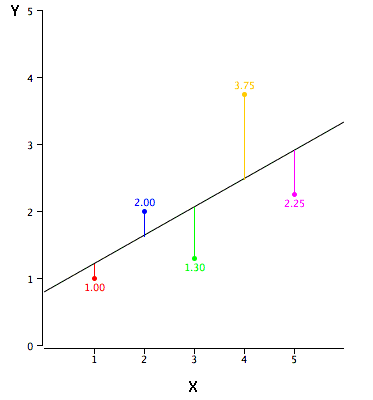1596969720

## Evaluation Metrics for Regression Problems

Hi, today we are going to study about the Evaluation metrics for regression problems. Evaluation Metrics are very important as they tell us, how accurate our model is.

Before we proceed to the evaluation techniques, it is important to gain some intuition.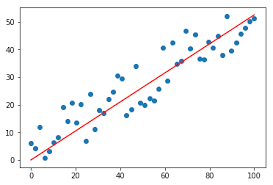In the above image, we can see that we have plotted a linear curve, but the curve is not perfect as some points are lying above the line & some are lying below the line.

So, how accurate our model is?

The evaluation metrics aim to solve these problems. Now, without wasting time, let’s jump to the evaluation metrics & see the evaluation techniques.

There are 6 evaluation techniques:

1. M.A.E (Mean Absolute Error)

2. M.S.E (Mean Squared Error)

3. R.M.S.E (Root Mean Squared Error)

4. R.M.S.L.E (Root Mean Squared Log Error)

5. R-Squared

Now, let’s discuss these techniques one by one.

### M.A.E (Mean Absolute Error)

It is the simplest & very widely used evaluation technique. It is simply the mean of difference b/w actual & predicted values.

Below, is the mathematical formula of the Mean Absolute Error.

Mean Absolute Error

The Scikit-Learn is a great library, as it has almost all the inbuilt functions that we need in our Data Science journey.

Below is the code to implement Mean Absolute Error

``````from sklearn.metrics import mean_absolute_error

mean_absolute_error(y_true, y_pred)
``````

Here, ‘y_true’ is the true target values & ‘y_pred’ is the predicted target values.

#artificial-intelligence #evaluation-metric #machine-learning #regression #statistics #deep learning1603022085

## Revisiting Regression Analysis

In Supervised Learning, we mostly deal with two types of variables i.e **numerical **variables and categorical variables. Wherein regression deals with numerical variables and **classification **deals with categorical variables. Where,

Regressionis one of the most popular statistical techniques used for Predictive Modelling and Data Mining in the world of Data Science. Basically,

Regression Analysis is a technique used for determining the relationship between two or more variables of interest.

However, Generally only 2–3 types of total 10+ types of regressions are used in practice. Linear Regression and Logistic Regression being widely used in general. So, Today we’re going to explore following 4 types of Regression Analysis techniques:

• Simple Linear Regression
• Ridge Regression
• Lasso Regression
• ElasticNet Regression

We will be observing their applications as well as the difference among them on the go while working on Student’s Score Prediction dataset. Let’s get started.

## 1. Linear Regression

It is the simplest form of regression. As the name suggests, if the variables of interest share a linear relationship, then Linear Regression algorithm is applicable to them. If there is a single independent variable(here, Hours), then it is a Simple Linear Regression. If there are more than 1 independent variables, then it is a Multiple Linear Regression. The mathematical equation that approximates linear relationship between independent (criterion ) variable X and dependent(predictor) variable Y is: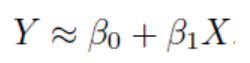where, β0 and β1 are intercept and slope respectively which are also known as parameters or model co-efficients.

#data-science #regression-analysis #elastic-net #ridge-regression #lasso-regression1594102200

# Introduction

In this article, we will analyse a business problem with linear regression in a step by step manner and try to interpret the statistical terms at each step to understand its inner workings. Although the liner regression algorithm is simple, for proper analysis, one should interpret the statistical results.

First, we will take a look at simple linear regression and after extending the problem to multiple linear regression.

For easy understanding, follow the python notebook side by side.

# What is Linear Regression?

Regression is the statistical approach to find the relationship between variables. Hence, the** Linear Regression** assumes a linear relationship between variables. Depending on the number of input variables, the regression problem classified into

1. Simple linear regression

2. Multiple linear regression

Let’s consider there is a company and it has to improve the sales of the product. The company spends money on different advertising media such as TV, radio, and newspaper to increase the sales of its products. The company records the money spent on each advertising media (in thousands of dollars) and the number of units of product sold (in thousands of units).

Now we have to help the company to find out the most effective way to spend money on advertising media to improve sales for the next year with a less advertising budget.

# Simple Linear Regression

Simple linear is an approach for predicting the quantitative response Y based on single predictor variable X.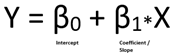This is the equation of straight-line having slope β1 and intercept β0.

Let’s start the regression analysis for given advertisement data with simple linear regression. Initially, we will consider the simple linear regression model for the sales and money spent on TV advertising media.

Then the mathematical equation becomes 𝑆𝑎𝑙𝑒𝑠 = 𝛽0 + 𝛽1 * 𝑇𝑉.

## Step 1: Estimating the coefficients: (Let’s find the coefficients)

Now to find the estimate of the sales for the advertising budget, we have to know the values of the β1 and β0. For the best estimate, the difference between predicted sales and the actual sales (called as residual) should be minimum.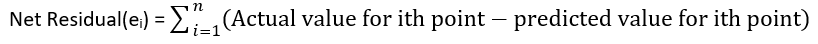As the residual may be negative or positive, so while calculating the net residual it can be lead to cancellation terms and reduction of net effect which leads to a non-optimal estimate of coefficients. To overcome this, we use a Residual sum of squares (RSS).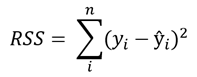With a simple calculation, we can find the value of β0 and β1 for minimum RSS value.

With the stats model library in python, we can find out the coefficients,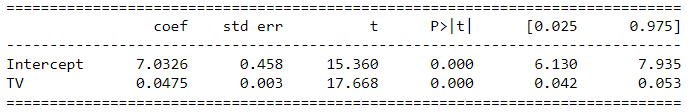Table 1: Simple regression of sales on TV

Values for β0 and β1 are 7.03 and 0.047 respectively. Then the relation becomes, Sales = 7.03 + 0.047 * TV.

This means if we spend an additional 1000 dollars on TV advertising media it increases the sales of products by 47 units.

This gives us how strongly the TV advertising media associated with the sales.

## Step 2: Assessing the Accuracy of the Coefficient Estimates ( How accurate these coefficients are? )

Why the coefficients are not perfect estimates?

The true relationship may not be perfectly linear, so there is an error that can be reduced by using a more complex model such as the polynomial regression model. These types of errors are called reducible errors.

On the other hand, errors may introduce because of errors in measurement and environmental conditions such as the office is closed for one week due to heavy rain which affects the sales. These types of errors are called**_ irreducible errors_**.

#linear-regression #machine-learning #basics #regression-analysis #data-science #data analysis1623856080

Have you ever visited a restaurant or movie theatre, only to be asked to participate in a survey? What about providing your email address in exchange for coupons? Do you ever wonder why you get ads for something you just searched for online? It all comes down to data collection and analysis. Indeed, everywhere you look today, there’s some form of data to be collected and analyzed. As you navigate running your business, you’ll need to create a data analytics plan for yourself. Data helps you solve problems , find new customers, and re-assess your marketing strategies. Automated business analysis tools provide key insights into your data. Below are a few of the many valuable benefits of using such a system for your organization’s data analysis needs.

#### Enhance marketing and ROI1604008800

## Static Code Analysis: What It Is? How to Use It?

Static code analysis refers to the technique of approximating the runtime behavior of a program. In other words, it is the process of predicting the output of a program without actually executing it.

Lately, however, the term “Static Code Analysis” is more commonly used to refer to one of the applications of this technique rather than the technique itself — program comprehension — understanding the program and detecting issues in it (anything from syntax errors to type mismatches, performance hogs likely bugs, security loopholes, etc.). This is the usage we’d be referring to throughout this post.

“The refinement of techniques for the prompt discovery of error serves as well as any other as a hallmark of what we mean by science.”

• J. Robert Oppenheimer

### Outline

We cover a lot of ground in this post. The aim is to build an understanding of static code analysis and to equip you with the basic theory, and the right tools so that you can write analyzers on your own.

We start our journey with laying down the essential parts of the pipeline which a compiler follows to understand what a piece of code does. We learn where to tap points in this pipeline to plug in our analyzers and extract meaningful information. In the latter half, we get our feet wet, and write four such static analyzers, completely from scratch, in Python.

Note that although the ideas here are discussed in light of Python, static code analyzers across all programming languages are carved out along similar lines. We chose Python because of the availability of an easy to use `ast` module, and wide adoption of the language itself.

### How does it all work?

Before a computer can finally “understand” and execute a piece of code, it goes through a series of complicated transformations: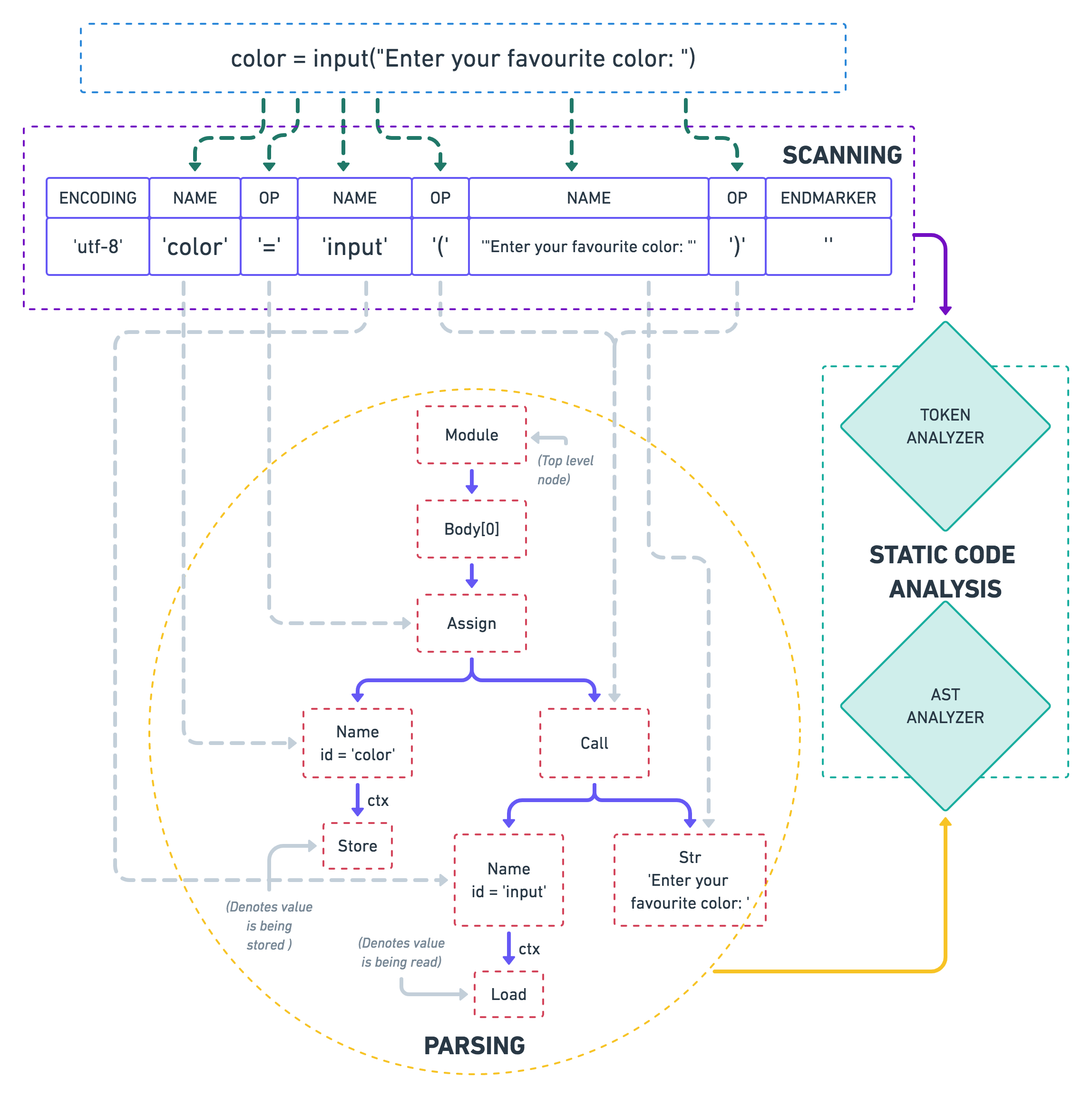As you can see in the diagram (go ahead, zoom it!), the static analyzers feed on the output of these stages. To be able to better understand the static analysis techniques, let’s look at each of these steps in some more detail:

### Scanning

The first thing that a compiler does when trying to understand a piece of code is to break it down into smaller chunks, also known as tokens. Tokens are akin to what words are in a language.

A token might consist of either a single character, like `(`, or literals (like integers, strings, e.g., `7``Bob`, etc.), or reserved keywords of that language (e.g, `def` in Python). Characters which do not contribute towards the semantics of a program, like trailing whitespace, comments, etc. are often discarded by the scanner.

Python provides the `tokenize` module in its standard library to let you play around with tokens:

Python

1

``````import io
``````

2

``````import tokenize
``````

3

4

``````code = b"color = input('Enter your favourite color: ')"
``````

5

6

``````for token in tokenize.tokenize(io.BytesIO(code).readline):
``````

7

``````    print(token)
``````

Python

1

``````TokenInfo(type=62 (ENCODING),  string='utf-8')
``````

2

``````TokenInfo(type=1  (NAME),      string='color')
``````

3

``````TokenInfo(type=54 (OP),        string='=')
``````

4

``````TokenInfo(type=1  (NAME),      string='input')
``````

5

``````TokenInfo(type=54 (OP),        string='(')
``````

6

``````TokenInfo(type=3  (STRING),    string="'Enter your favourite color: '")
``````

7

``````TokenInfo(type=54 (OP),        string=')')
``````

8

``````TokenInfo(type=4  (NEWLINE),   string='')
``````

9

``````TokenInfo(type=0  (ENDMARKER), string='')
``````

(Note that for the sake of readability, I’ve omitted a few columns from the result above — metadata like starting index, ending index, a copy of the line on which a token occurs, etc.)

#code quality #code review #static analysis #static code analysis #code analysis #static analysis tools #code review tips #static code analyzer #static code analysis tool #static analyzer